# Find the center, vertices and foci of the hyperbola.x22536a. Center: (0, 0)(0, 6)(0, /61)Vertices:Foci:b. Center: (0, 0)(t6, 0)Vertices:Foci: (+v61, 0)c. Center: (0, 0)Vertices: (+6, 0)(61, 0)Foci:d. Center: (0, 0)(6, 0)Vertices:Foci: (+V61, 0)e. Center: (0, 0)(0, +5)Vertices:(0, ±/61)Foci:

Question
1 views

I am needing assistance with the attached equation.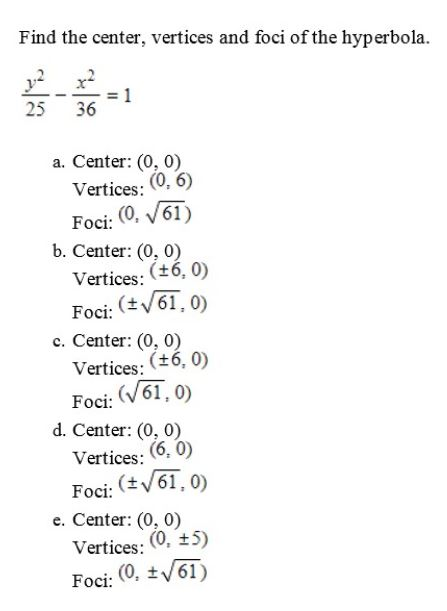help_outlineImage TranscriptioncloseFind the center, vertices and foci of the hyperbola. x2 25 36 a. Center: (0, 0) (0, 6) (0, /61) Vertices: Foci: b. Center: (0, 0) (t6, 0) Vertices: Foci: (+v61, 0) c. Center: (0, 0) Vertices: (+6, 0) (61, 0) Foci: d. Center: (0, 0) (6, 0) Vertices: Foci: (+V61, 0) e. Center: (0, 0) (0, +5) Vertices: (0, ±/61) Foci: fullscreen
check_circle

Step 1

Given: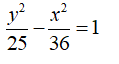General equation of hyperbola centred at (h,k) and vertical transverse axis is given as: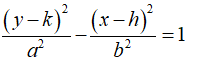Step 2

Rewriting and comparing the given equation, we get: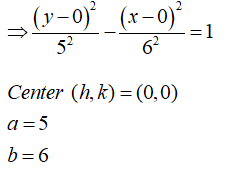Vertices of the hyperbola are given as: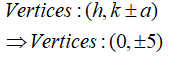Step 3

Now,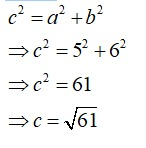Hence focus of hyperbola is given as: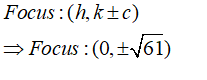...

### Want to see the full answer?

See Solution

#### Want to see this answer and more?

Solutions are written by subject experts who are available 24/7. Questions are typically answered within 1 hour.*

See Solution
*Response times may vary by subject and question.
Tagged in